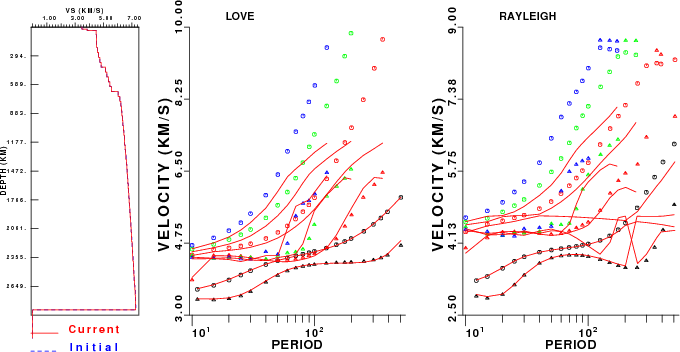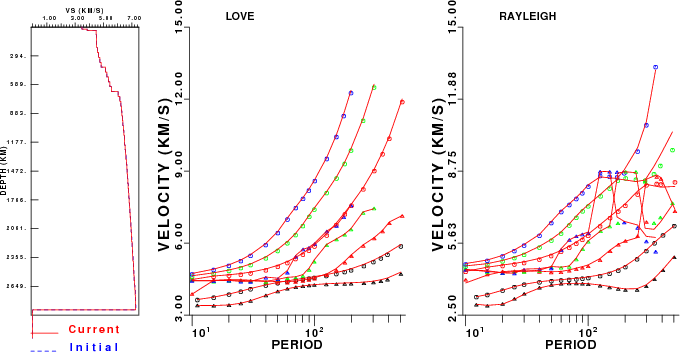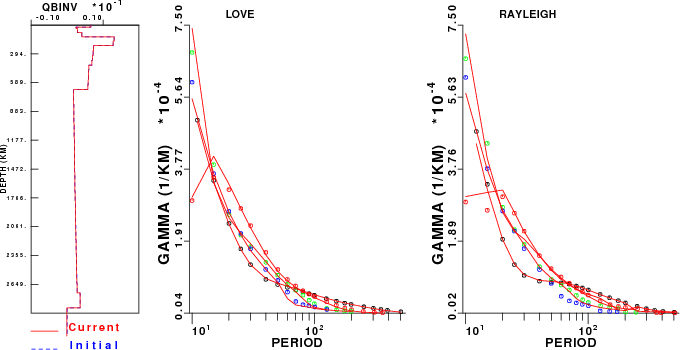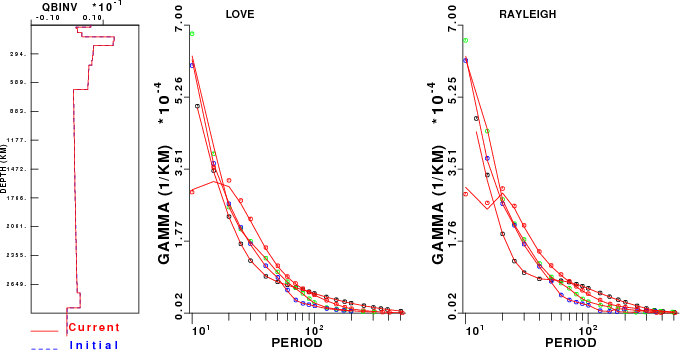## Earth flattening - surface-wave dispersion

### Introduction

In 2004, David Harkrider investigated the appropriateness of the Earth-flattening approximation by comparing the phase velocities from minos to those from his plane-layer dispersion codes.  To make the comparison easier, he replaced the l order parameter in minos  by a float, so that he could get the effective free oscillation dispersion at periods such as 100 or 200 seconds.  He restricted his comparison to the dispersion for infinite-Q models

The objective here is to apply the concept of his comparison to the same model and transformations used for the waveform comparison.  In doing we wish to check the flattening approximation used in the dispersion inversion program surf96, which is a newer version of David Russell's 1985 version.  The flattening approximation of Russell is based on the work of Schwab and Knopoff (1972). The primary difference here is that we use the Biswas (1972) density mapping (PAGEOPH 96, 61-74, 1972) and that we do not use the average layer velocity of Schwab and Knopoff (1972) (Methods of Computational Physics, Vol 12).

We use the surf96 program for the comparison because that program permits the computation for the effects of causal Q on the group velocity dispersion. We will also compare the spatial attenuation values gamma.

The first step was to convert from the mineos output

n t   l      C(km/s)         f(mHz)          T(sec)          U(km/s)          Q
0 s  999    3.277979        81.84677        12.21795        2.926002        185.5879      -0.4165177E-02

to the surf96 dispersion format:

`SURF96 R C X 0 12.21795 3.277979 0.0010SURF96 R U X 0 12.21795 2.926002 0.0010SURF96 R G X 0 12.21795  4.73508e-04 1.00000e-08`
The conversion is straight forward. However to estimate gamma we use the Aki and Richard (2002) definition (equations 7.03/7.04) to give gamma = pi / Q U T .

Because the surf96 code only permits a fluid layer at the top of the model, we approximate the effect of the liquid core by replacing the outer core with a single layer with model96 parameters

`    47.8301     8.0191     0.0010     9.9543  0.578E+05   0.00       0.00       0.00       1.00       1.00`
and then modified the surface-wave disperesion and inversion codes to use that false S-velocity for the dispersion relation, but not to use that low velocity in the root search. By adding this single layer, the higher modes are fit better at the longer periods. This model is called nntak135sph.mod.

The plane-layer  dispersion computations do not account for gravity.  The free-oscillation computations include the effect of gravity up to frequencies of 10.0 mHz. We did not check the effect for higher frequencies.

Fri Dec 28 13:53:41 CST 2007: I modified srfdis96, srfdrl96, srfdrr96, sdisp96, sregn96 and slegn96 to permit a fluid core by specifiying the shear-wave velocity in the core as 0.001 km/s. The logic for selecting the phase velocity search window assumes that this is a fluid and only uses the P-velocity as a guide. The test is made on whether the transformed velocity is > 0.01 km/sec, e.g.,

`        do 20 i=1,mmax        if(b(i).gt.0.01 .and. b(i).lt.betmn)then            betmn = b(i)            jmn = i            jsol = 1        elseif(b(i).le.0.01 .and. a(i).lt.betmn)then            betmn = a(i)            jmn = i            jsol = 0        endif        if(b(i).gt.betmx) betmx=b(i)   20 continue      WRITE(6,*)'betmn, betmx:',betmn, betmx`
The Love wave eigenfunction program ignores the low S-velocity, but the boundary condition of a stress-free boundary is correctly applied.  The advantage of this subterfuge is that the effects of a fluid core on the Rayleigh dispersion can be seen.

To perform the comparison, I go to the directory MINEOS/share/mineos1/nnDEMO6 (this appears when the MINEOS.tgz is unpacked), create the free oscillation synthetics
RUN_MINEOS.sh tak135-f

then I run DOSEL to make the comparison, which polulates the MINEOS/sahre/mineos1/HTML.SW with graphics, and finally  I run DOCLEAN to clean up the directory.

Using the command  surf96 1 17  (17.txt) we can compare the observed (mineos) dispersion (and the surface-wave predicted dispersion with the current flattening model) at the long periods:

`       Mode        Period       Observed      Predicted  L C    0    301.7149963      5.2029619      5.2837887       L C    0    347.5029907      5.3578548      5.4408402       L C    0    392.8059998      5.5085521      5.5924311       L C    0    506.1719971      5.8580852      5.9402952       L U    0    301.7149963      4.3387032      4.4140792       L U    0    347.5029907      4.3883481      4.4704652       L U    0    392.8059998      4.4559240      4.5439758       L U    0    506.1719971      4.6907072      4.7881312       R C    0    297.7600098      5.2720690      5.3223057       R C    0    347.7680054      5.6149321      5.6543474       R C    0    407.5010071      5.9535398      5.9821863       R C    0    503.4719849      6.3606620      6.3766308       R U    0    297.7600098      3.7275290      3.8109736       R U    0    347.7680054      4.0285168      4.1075196       R U    0    407.5010071      4.4197330      4.4858279       R U    0    503.4719849      4.9883242      5.0503368      `
The interesting part of this comparison is that if we wish to fit observations in the time domain to with 4 sec at a 90 degree epicentral distance, we would require a
precision better than 4/ 4000, or 0.1%.  The dispersion comparison here shows deviations  of 2%.  Of course this difference also reflects the use of the Schwab and Knopoff adjustment factor of

c = c / tm
u = u * tm
where
tm = sqrt(1.+(3.*c/(2.*a*om))**2)  [a is the radius of the Earth and om is the angulat frequency] for Love
and
tm = sqrt(1.+(c/(2.*a*om))**2)  for Rayleigh.
Note that the wavenumber integration synthetics do not have such a factor!

### Dispersion Comparison

We compute the dispersion for  two models - the spherical model with flattening, e.g., with the keyword SPHERICAL EARTH, and the same model treated as a flat-Earth model, e.g., replacing the SPHERICAL EARTH keywork with FLAT EARTH in the file nntak135sph.mod. The observed (mineos) and surf96 predicted dispersion are in the file 17.txt.

 No flattening FlatteningComparison of non-flattened dispersion predictions (solid curves) to free oscillation values (symbols). Phase velocity is indicated by circle and the group velocity by a triangle. Modes: fundamental is black, 1'st is red,  2'nd is green and 3'rd is blue. Comparison of Earth-flattening dispersion predictions (solid curves) to free oscillation values (symbols). Phase velocity is indicated by circle and the group velocity by a triangle. Modes: fundamental is black, 1'st is red, 2'nd is green and 3'rd is blue.

The difference in the use of flattening instead of no-flattening is especially noticeable in the higher modes. At periods greater than 300 seconds, the differences are noticeable even in the fundamental mode.

### Gamma Comparison

The observed (mineos) and surf96 predicted gamma dispersion are in the file 12.txt.

 No flattening FlatteningComparison of non-flattened gamma predictions (solid curves) to free oscillation values (symbols). Modes: fundamental is black, 1'st is red, 2'nd is green and 3'rd is blue. Comparison of Earth-flattening gamma predictions (solid curves) to free oscillation values (symbols).  Modes: fundamental is black, 1'st is red, 2'nd is green and 3'rd is blue.

The results of the gamma comparison indicates that the differences are small.  One reason is that the gamma is defines as pi f / Q U T, and the values are quite small at long period.

Last Changed February 4, 2008